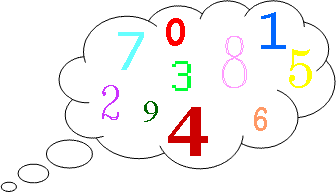### Submit a Resource

NRICH: Think of Two Numbers

Think of two whole numbers under 10, and follow the steps. I can work out both your numbers very quickly. How?

Here is an alternative and more unusual version of the “Think of a Number” trick which you may have heard of before.Think of two whole numbers under 10.

Take one of them and add 1.
Multiply by 5.
Subtract 1.

Double again.
Subtract 8.
Halve this number and tell me your answer.

From your answer I can work out both your numbers very quickly. How?

Choose some different pairs of numbers and repeat the process.
Can you figure out how the trick works?

Age 11 to 14

Math Topics
Algebra & Pre-Algebra, Linear Equations
Middle School, High School, Educator
Descriptions of PDFs

Click here for a poster of this problem.

## Organization

NRICH (University of Cambridge)

Challenge
PDF File